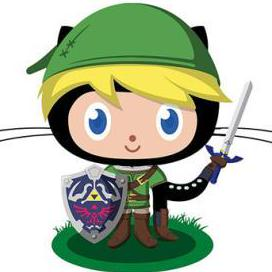为啥等于0啊，不等于20？

#include<stdio.h>

int main(void) {
int a,b,t;
t=0;
scanf("%d,%d",&a,&b);
if(a>b) t=a;a=b;b=t;
printf("%d",b);
return 0;
}


a=b
b=t

2

1

1

1

1

b=t不受if控制，一定会执行（t又是0），彻底把b置成0了。

1

if(a>b)
t=a;
a=b;
b=t;


if(a>b)
{
t=a;
a=b;
b=t;
}